# Thank you for registering for our upcoming Dirty Duathlon or Trail Run, presented by Northside Hospital - Cherokee, at Rope Mill Park on November 11th. The following outlines the procedures that will be implemented for the 2023 edition of this event. Knowing these procedures will help us ensure we have a safe and smooth event for everyone.

 /* styles */ If you need to make changes such as a new teammate or category, please email me now and get that taken care of. No changes after Monday November 6th.
 /* styles */ If you come to the optional Thursday night check in, bring this waiver with you. A signed waiver is needed to pick up your race number and swag. We have a bonus swag item for the first 250 to check in, so come see us at the Thursday night check in on November 9th from 4-7 pm at OutSpokin Bicycles, 3422 Sixes Rd #110, Canton, GA 30114
 /* styles */ You must start in your assigned start wave, which is by category. All riders in each wave receive the same start time (gun time).
 table div table+table+table+table+table+table+table+table+table+table div table{width:100%;padding:0}table div table+table+table+table+table+table+table+table+table+table div table img{width:96.23%;padding:0;float:none}table div table+table+table+table+table+table+table+table+table+table div table td{width:100%;padding:0 1.88% 18px}/* styles */# CHECK-IN

## OPTIONAL early packet pickup is at OUTSPOKIN BICYCLES on THURSDAY 11/9 from 4 to 7 pm. 3422 Sixes Rd #110, Canton, GA 30114

You will receive your race number and swag at this time. If you check in early, you can go straight to the start on Saturday morning. (BRING SIGNED WAIVER TO EARLY CHECK-IN)

▪ Saturday Check-in hours are from 7 am to 8:30 am.
▪ Waivers should be printed at home, filled out ahead of time, and brought to packet pickup. CLICK HERE FOR EVENT WAIVER You may pick up someone else's number ONLY IF YOU BRING A WAIVER SIGNED BY THEM.
▪ No race day registrations or participant swaps. If you want to change teammates, categories or need to switch from team to solo, you need to contact me via email no later than 11/6 and we'll get you taken care of. No changes after 11/6.
▪ When you arrive, parking volunteers will direct you where to park, which will be in one of 3 locations depending on when you arrive. If you arrive later, be prepared to park at the Kirk Rudy office and jog/ride the 1/2 mile down the road to the start. Leave yourself enough time to check-in and get to the start. We will have a bag drop area at the pavilion for trail run only participants or you can leave items in the transition area so you do not have to go back to your car until after the race.
 ▪ Saturday Check-in hours are from 7 am to 8:30 am.
 ▪ Waivers should be printed at home, filled out ahead of time, and brought to packet pickup. CLICK HERE FOR EVENT WAIVER You may pick up someone else's number ONLY IF YOU BRING A WAIVER SIGNED BY THEM.
 ▪ No race day registrations or participant swaps. If you want to change teammates, categories or need to switch from team to solo, you need to contact me via email no later than 11/6 and we'll get you taken care of. No changes after 11/6.
 ▪ When you arrive, parking volunteers will direct you where to park, which will be in one of 3 locations depending on when you arrive. If you arrive later, be prepared to park at the Kirk Rudy office and jog/ride the 1/2 mile down the road to the start. Leave yourself enough time to check-in and get to the start. We will have a bag drop area at the pavilion for trail run only participants or you can leave items in the transition area so you do not have to go back to your car until after the race.

# RACE PROCEDURES

▪ We will have a brief pre-race meeting at 8:35 am before staging starts.
▪ RACERS ONLY in the transition area. It wil be crowded in the Transition Area, especially pre-race as racers will be setting up their gear. Please have your family members/spectators hang out elsewhere to reduce crowding.
▪ Make sure the tyvek race bib is pinned on your FRONT. Those in the trail run only will have a tyvek bib with timing chip. Those in the Duathlon will NOT have a timing chip on their running bib. All duathlon timing is done with the bike number plate, which gets mounted on your handlebars. If you are a team in the duathlon, the rider gets the bike plate and the runner wears the pin-on tyvek race number. Solo duathlon participants wear the pin-on tyvek race number as well as mount the number plate to their bike handlebars.
▪ If you are on a 3 person team, you will need to transfer the bike plate to the second rider's bike while your runner is completing the run segment.
▪ We will have multiple start waves for the trail run and duathlon beginning at 8:58 am. YOU CAN VIEW YOUR START WAVE HERE. Your wave number will be written on your number plate.
▪ There is an Aid Station with water, Hammer HEED and Hammer Gels on the run course at approximately mile 1 and mile 2, so you shouldn't need to carry anything on the run.
▪ Do not litter anywhere on the course - penalty is a DQ
▪ Course will be well marked with pink ribbons and pink/black arrow signs at turns. Pink ribbons indicate the direction you want to head and are also used to designate that you are on-course if it has been a while in between turns. Course marshals will be positioned throughout the course for your safety.
▪ Duathlon course is bike the Mill Trails CW, run Explorer Trails CCW, then bike Avalanche Trails CCW. The 3.3 Mile Trail Run will run the Explorer Trails CCW as well.
▪ Announce if you need to pass runners/riders ahead by saying NEED TO PASS, RIDER BACK or ON YOU LEFT/RIGHT and give them a chance to move over a bit BEFORE YOU PASS THEM. It is the passing riders responsibility to execute a clean pass. BE NICE TO EACH OTHER and work together,as passing can be tricky on the Avalanche Trail.
▪ Snacks and drinks will be available for all participants at the pavilion post-race.
▪ Port-o-lets as well as permanent bathrooms with running water will be available at this venue.
 ▪ We will have a brief pre-race meeting at 8:35 am before staging starts.
 ▪ RACERS ONLY in the transition area. It wil be crowded in the Transition Area, especially pre-race as racers will be setting up their gear. Please have your family members/spectators hang out elsewhere to reduce crowding.
 ▪ Make sure the tyvek race bib is pinned on your FRONT. Those in the trail run only will have a tyvek bib with timing chip. Those in the Duathlon will NOT have a timing chip on their running bib. All duathlon timing is done with the bike number plate, which gets mounted on your handlebars. If you are a team in the duathlon, the rider gets the bike plate and the runner wears the pin-on tyvek race number. Solo duathlon participants wear the pin-on tyvek race number as well as mount the number plate to their bike handlebars.
 ▪ If you are on a 3 person team, you will need to transfer the bike plate to the second rider's bike while your runner is completing the run segment.
 ▪ We will have multiple start waves for the trail run and duathlon beginning at 8:58 am. YOU CAN VIEW YOUR START WAVE HERE. Your wave number will be written on your number plate.
 ▪ There is an Aid Station with water, Hammer HEED and Hammer Gels on the run course at approximately mile 1 and mile 2, so you shouldn't need to carry anything on the run.
 ▪ Do not litter anywhere on the course - penalty is a DQ
 ▪ Course will be well marked with pink ribbons and pink/black arrow signs at turns. Pink ribbons indicate the direction you want to head and are also used to designate that you are on-course if it has been a while in between turns. Course marshals will be positioned throughout the course for your safety.
 ▪ Duathlon course is bike the Mill Trails CW, run Explorer Trails CCW, then bike Avalanche Trails CCW. The 3.3 Mile Trail Run will run the Explorer Trails CCW as well.
 ▪ Announce if you need to pass runners/riders ahead by saying NEED TO PASS, RIDER BACK or ON YOU LEFT/RIGHT and give them a chance to move over a bit BEFORE YOU PASS THEM. It is the passing riders responsibility to execute a clean pass. BE NICE TO EACH OTHER and work together,as passing can be tricky on the Avalanche Trail.
 ▪ Snacks and drinks will be available for all participants at the pavilion post-race.
 ▪ Port-o-lets as well as permanent bathrooms with running water will be available at this venue.

# COURSE FLOW/TRANSITION AREA SETUP

Solos: ensure your pin-on number is pinned to the front of your body and the cardboard number is mounted on the handlebar of your bike. Teams: ensure pin-on number is pinned on front of RUNNER, and platic number is mounted on handlebar of bike for RIDER. 3 Person Teams: ensure you switch the bike number to the second rider's bike while your runner is out.

Duathlon participants will start the race on bike. There will be start corrals marked along the concrete path that correspond to your start wave. You need to exit the Transition Area by 8:45 and head to start staging. YOU CAN VIEW YOUR START WAVE HERE. Your wave number will be written on your number plate. You will be released in waves and complete the Mill Trails CW.

As riders return from Bike Leg 1 on the Mill Trails, you will need to dismount your bike in the grass just past the bridge and run through the small ditch into the Transition Area. There will be signs that say DISMOUNT HERE. You must dismount and run your bike into the TA.

Once in the transition area, solos will rack their bike and then head out the back of the TA onto Explorer and complete the run loop CCW. For teams, the rider needs to physically tag their runner before the runner can head out. Teams should also re-rack their bikes while waiting. You must keep the aisles of the TA clear - do not block aisles with bikes,and try to stay out of the way of your bike rack neighbors while waiting.

Once back from the run, solos retrieve their bike from the racks and they need to RUN it out of the TA (no riding in TA) across the small wooden bridge, back towards the finish arch. Once across the bridge, you can get back on the bike and ride through the finish arch, through the circle parking lot and onto Avalanche Trail CCW (This will all be marked). For teams, the returning runner needs to tag the rider before the rider can leave the TA, also RUNNING their bike across the wooden bridge. The main aisle of the TA leading to the wooden bridge needs to be kept clear so Team riders should not unrack their bike until they see their runner heading back in.

Once riders complete bike leg 2 on Avalanche CCW, they will finish via the big hill behind the pavilion and ride through the finish. This hill will be leaf blown and kudzu pruned back before the race for your safety.

No spectators in the TA - please encourage them to watch elsewhere.

Once you finish the race, you may not exit TA over the wooden bridge when done as you will interfere with finishing traffic. You must exit out the back of TA or through the woods on the side.

# RESULTS/AWARDS

▪ Finish times wlll be uploaded in real time to tablets that we will have at our results tent, so check your finish time and placing. Report any errors to Chris Randall at timing.
▪ Trail Run awards are at 10:15 am Duathlon awards will be at approximately 11:15 am, assuming we have enough finishers in. AWARDS WILL NOT BE MAILED - THEY MUST BE PICKED UP AT THE RACE
 ▪ Finish times wlll be uploaded in real time to tablets that we will have at our results tent, so check your finish time and placing. Report any errors to Chris Randall at timing.
 ▪ Trail Run awards are at 10:15 am Duathlon awards will be at approximately 11:15 am, assuming we have enough finishers in. AWARDS WILL NOT BE MAILED - THEY MUST BE PICKED UP AT THE RACE
 table div table+table+table+table+table+table+table+table+table+table+table+table+table div table{width:100%;padding:0}table div table+table+table+table+table+table+table+table+table+table+table+table+table div table img{width:96.23%;padding:0;float:none}table div table+table+table+table+table+table+table+table+table+table+table+table+table div table td{width:100%;padding:0 1.88% 18px}/* styles */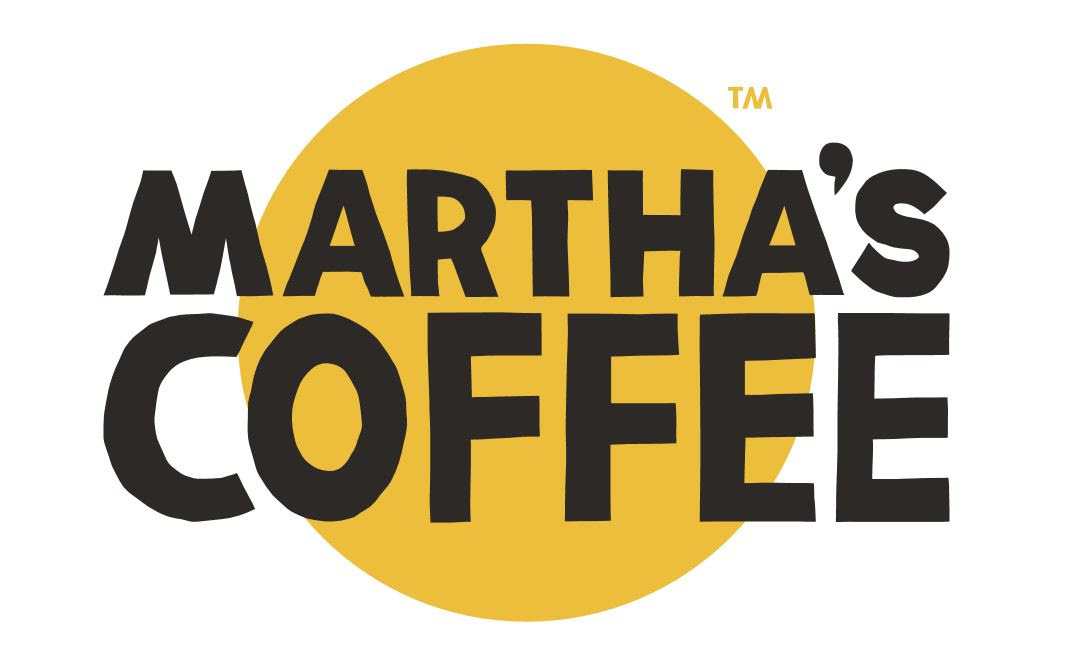Marthas Outpost will be on-site for the event. They will have coffee, tea and hot chocolate available for purchase so you can stay warm on race morning! They typically set up in the parking lot to the left as you enter the park.

 table div table+table+table+table+table+table+table+table+table+table+table+table+table+table+table div table{width:100%;padding:0}table div table+table+table+table+table+table+table+table+table+table+table+table+table+table+table div table img{width:96.23%;padding:0;float:none}table div table+table+table+table+table+table+table+table+table+table+table+table+table+table+table div table td{width:100%;padding:0 1.88% 18px}/* styles */## 2023 Goat Gear! Cash or Venmo on Race Day!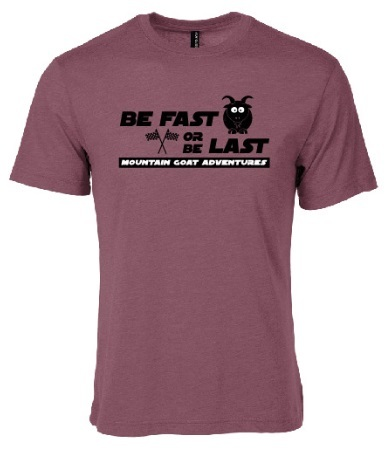We have an entire box of leftover event shirts from 2023 that we will have available at this event. Help us clear them out and take a few home. These are 2 for \$5. We have both men's and ladies sizes available in various race designs.

Our newest casual shirt: "Be Fast or Be Last" is available in many colors, in sizes XS - 2XL for \$15 each.

We also still have a few hooded sweathshirts left and are selling these for \$25 each.

We will also have our run hats, visors and thermal beanies for \$15 each. Trucker Caps are \$20 each.

Cash or Venmo accepted on merchandise sales.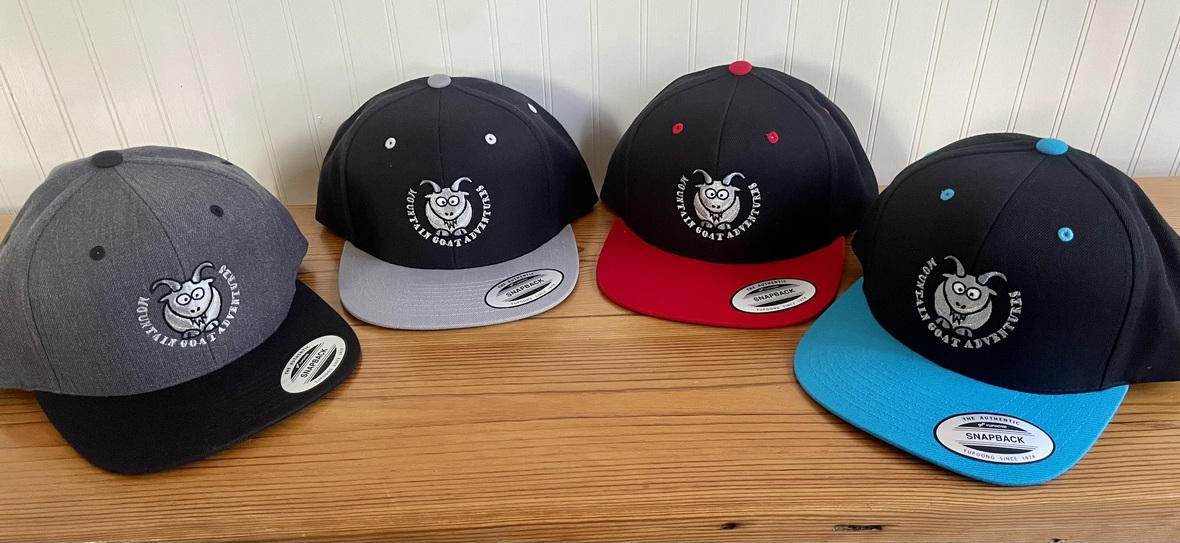table div table+table+table+table+table+table+table+table+table+table+table+table+table+table+table+table+table+table+table div table{width:100%;padding:0}table div table+table+table+table+table+table+table+table+table+table+table+table+table+table+table+table+table+table+table div table img{width:96.23%;padding:0;float:none}table div table+table+table+table+table+table+table+table+table+table+table+table+table+table+table+table+table+table+table div table td{width:100%;padding:0 1.88% 18px}/* styles */## Hammer Nutrition Race Day Fueling Tips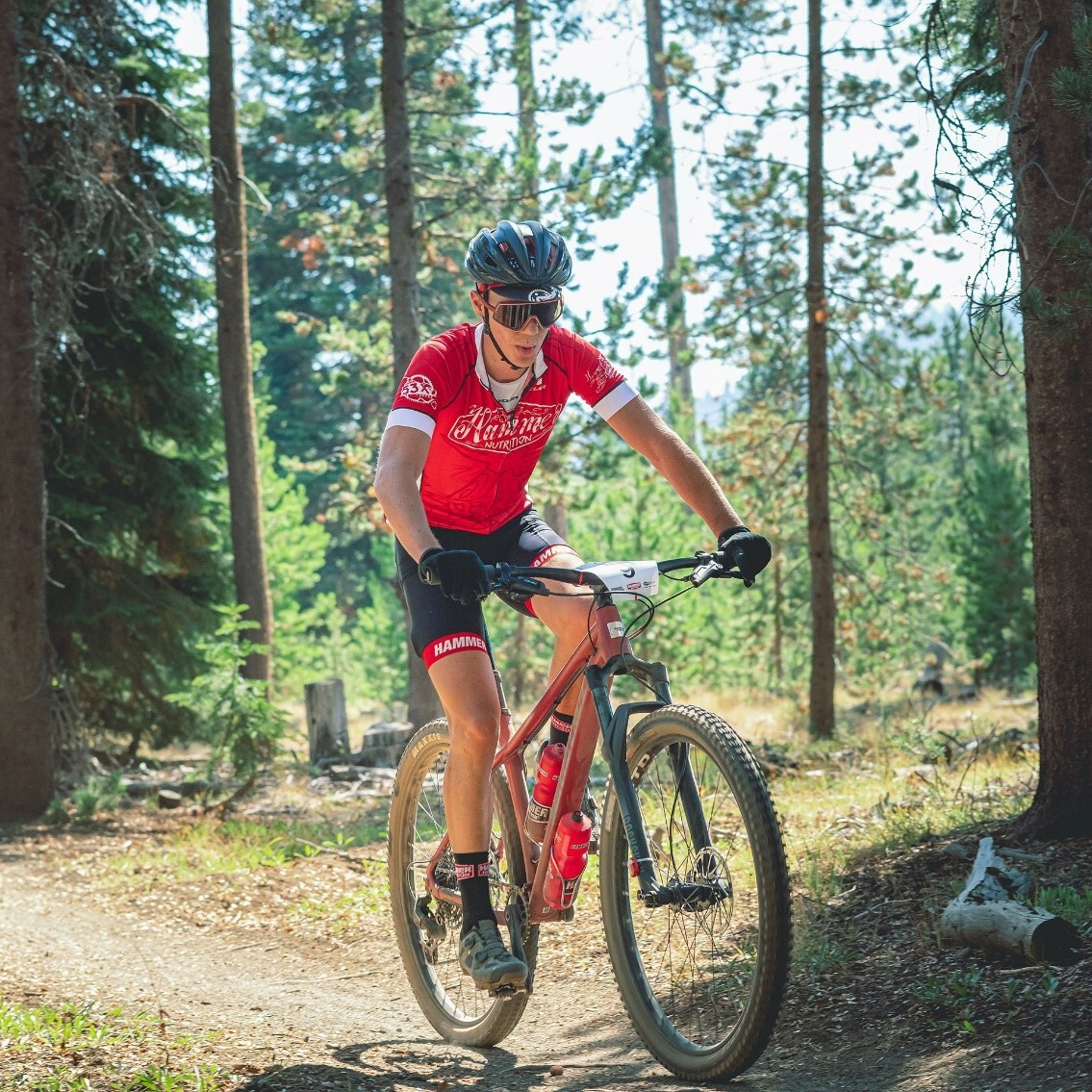Hammer Nutrition endurance fuels will be on the course at the 2023 Rope Mill Duathlon and Trail Run! Hammer’s been supplying athletes with natural products free of added sugars, artificial colors, flavors, chemicals, and preservatives for over 30 years.

## ROPE MILL RACE TIP #1: REPLENISH ELECTROLYTES WITH A BALANCED FORMULA (NOT JUST SALT!) IN AMOUNTS APPROPRIATE FOR CONDITIONS

FACT: Salt (sodium chloride) cannot fulfill your entire requirements for electrolytes. The minerals calcium, magnesium, and potassium also must be replenished to ensure the proper functioning of key body systems. In addition, your daily dietary sodium intake, fitness level, acclimatization, and the environmental conditions (heat, humidity, etc.) all affect the number of electrolytes you will need to replenish during exercise.

For a balanced, full-spectrum formula of electrolytes, replenish with Endurolytes. Choose regular Endurolytes, Endurolytes Extreme, or Endurolytes Fizz in doses appropriate for the conditions.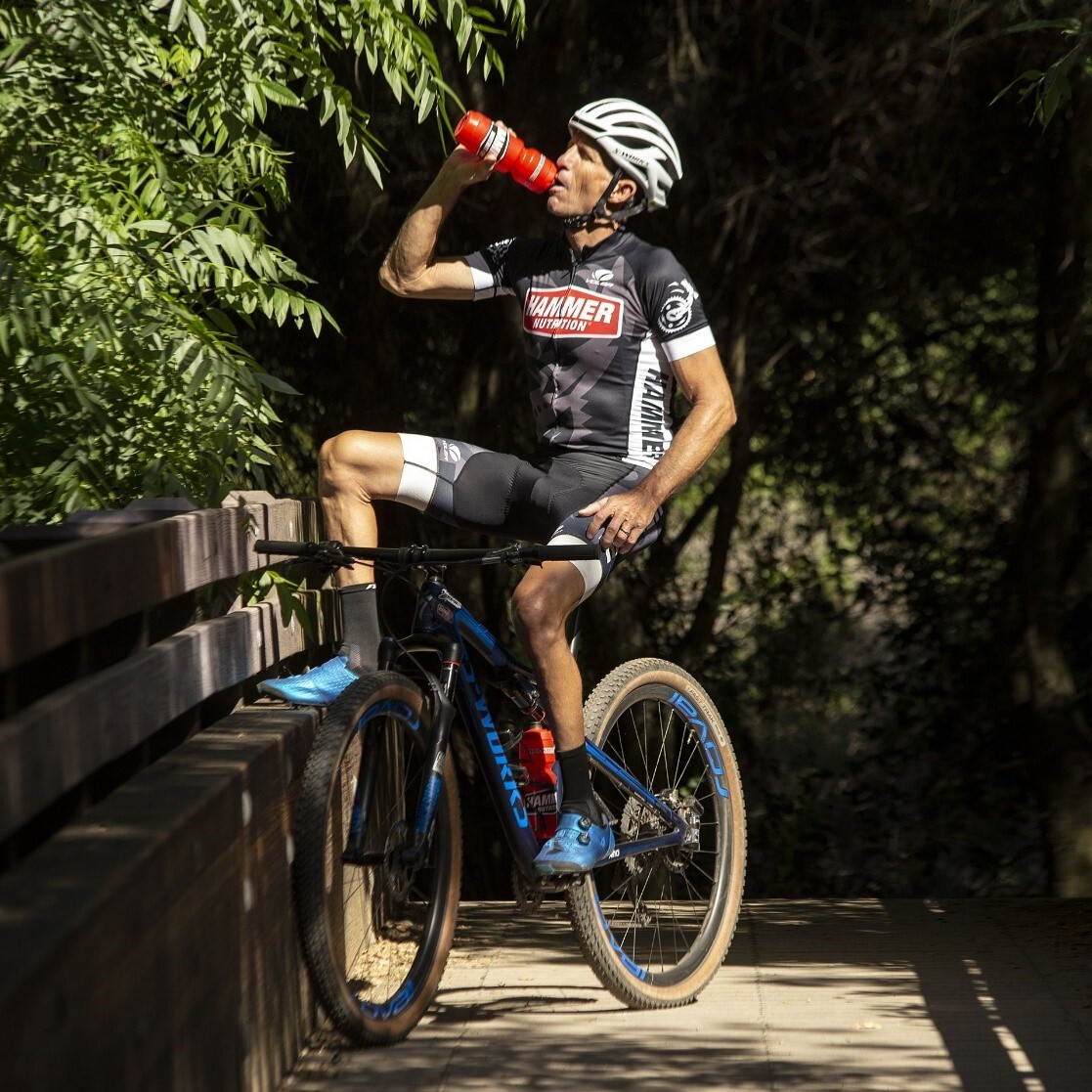## ROPE MILL RACE TIP #2: BEFORE WORKOUTS AND RACES, CONSUME 300-400 CALORIES. COMPLETE YOUR MEAL 3 HOURS BEFORE YOU START EXERCISE

To perform and feel your best during races or workouts, consume no more than 300-400 calories. Choose easily digested, complex carbohydrates, along with a small amount of protein and a little healthy fat. Avoid fiber, simple sugar, and acidic foods. Finish your meal 3 hours before exercise. Eating within 3 hours can seriously hurt your performance by 1) reducing the conversion of fats to fuels, and 2) accelerating glycogen depletion.

Tip: If your race is early, don't sacrifice sleep to eat! Instead, consume a small amount of supplemental fuel, such as one Hammer Gel, about 5 minutes before starting.

 table div table+table+table+table+table+table+table+table+table+table+table+table+table+table+table+table+table+table+table+table+table+table+table div table{width:100%;padding:0}table div table+table+table+table+table+table+table+table+table+table+table+table+table+table+table+table+table+table+table+table+table+table+table div table img{width:96.23%;padding:0;float:none}table div table+table+table+table+table+table+table+table+table+table+table+table+table+table+table+table+table+table+table+table+table+table+table div table td{width:100%;padding:0 1.88% 18px}/* styles */## Volunteer Opportunities and Trail Work Parties!

This event is one of four major yearly fundrasisers for SORBA Woodstock. Thanks to generous support we receive from Northside Hospital-Cherokee and YOUR PARTICIPATION, this event raises roughly \$2500 for the chapter! Thank you for supporting this event and your local trails!

## Want to help work on your local trails?

Visit the SORBA Woodstock Website and view the calendar for work party dates and locations. Wear long pants and closed-toe shoes, and bring your own water. SORBA Woodstock provides the rest. This is a chance to help give back to the trails. SORBA Woodstock could not maintain our wonderful trails without the help of a small army of generous volunteers like you!

 table div table+table+table+table+table+table+table+table+table+table+table+table+table+table+table+table+table+table+table+table+table+table+table+table+table+table div table{width:100%;padding:0}table div table+table+table+table+table+table+table+table+table+table+table+table+table+table+table+table+table+table+table+table+table+table+table+table+table+table div table img{width:96.23%;padding:0;float:none}table div table+table+table+table+table+table+table+table+table+table+table+table+table+table+table+table+table+table+table+table+table+table+table+table+table+table div table td{width:100%;padding:0 1.88% 18px}/* styles */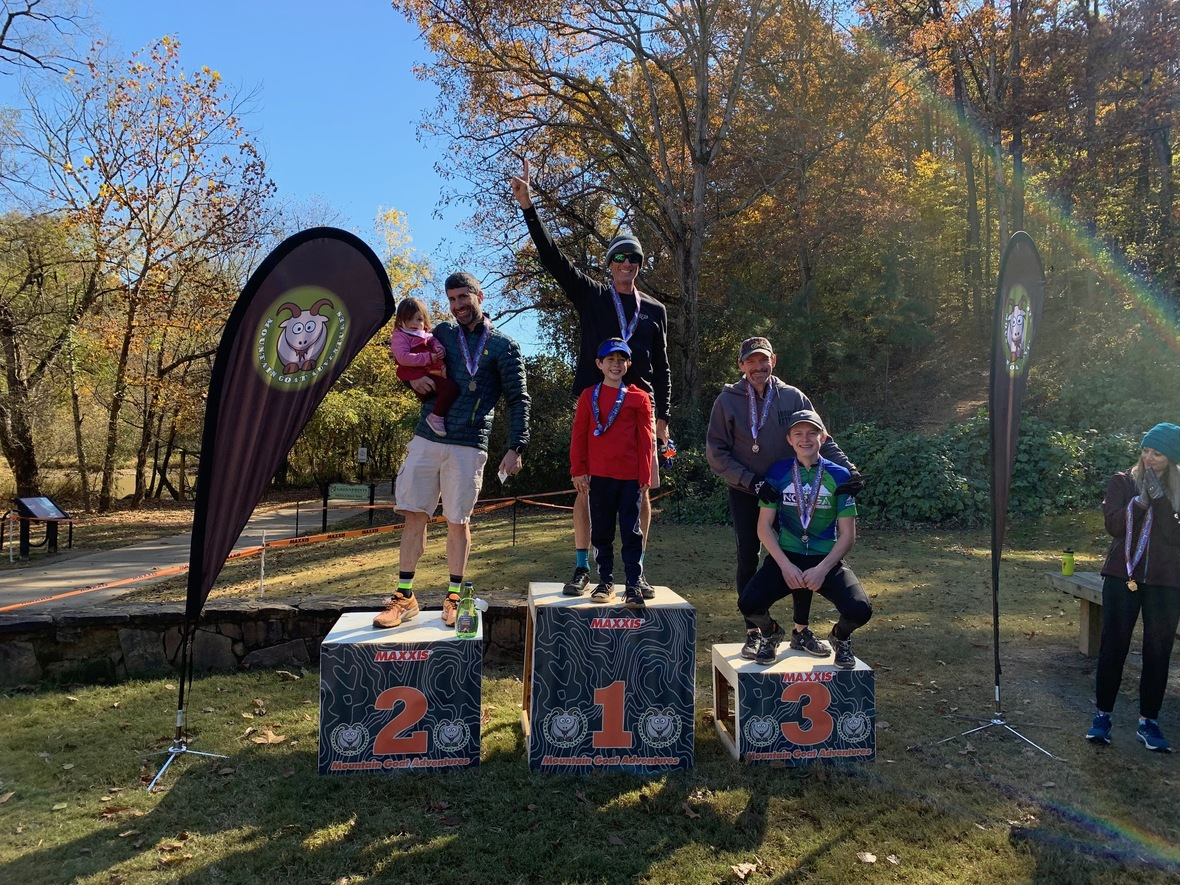table div table+table+table+table+table+table+table+table+table+table+table+table+table+table+table+table+table+table+table+table+table+table+table+table+table+table+table+table div table{width:100%;padding:0}table div table+table+table+table+table+table+table+table+table+table+table+table+table+table+table+table+table+table+table+table+table+table+table+table+table+table+table+table div table img{width:96.23%;padding:0;float:none}table div table+table+table+table+table+table+table+table+table+table+table+table+table+table+table+table+table+table+table+table+table+table+table+table+table+table+table+table div table td{width:100%;padding:0 1.88% 18px}/* styles */## Special Thanks to our SORBA Woodstock Sponsors!

 table div table+table+table+table+table+table+table+table+table+table+table+table+table+table+table+table+table+table+table+table+table+table+table+table+table+table+table+table+table+table div table{width:100%;padding:0}table div table+table+table+table+table+table+table+table+table+table+table+table+table+table+table+table+table+table+table+table+table+table+table+table+table+table+table+table+table+table div table table{padding:0;float:left!important;width:30.566%!important}table div table+table+table+table+table+table+table+table+table+table+table+table+table+table+table+table+table+table+table+table+table+table+table+table+table+table+table+table+table+table div table table+table td,table div table+table+table+table+table+table+table+table+table+table+table+table+table+table+table+table+table+table+table+table+table+table+table+table+table+table+table+table+table+table div table td{padding-left:0;padding-right:0}table div table+table+table+table+table+table+table+table+table+table+table+table+table+table+table+table+table+table+table+table+table+table+table+table+table+table+table+table+table+table div table table td{padding-left:0;padding-right:20px}table div table+table+table+table+table+table+table+table+table+table+table+table+table+table+table+table+table+table+table+table+table+table+table+table+table+table+table+table+table+table div table table+table{float:left!important;width:69.434%!important}/* styles */
 table div table+table+table+table+table+table+table+table+table+table+table+table+table+table+table+table+table+table+table+table+table+table+table+table+table+table+table+table+table+table+table div table{width:100%;padding:0}table div table+table+table+table+table+table+table+table+table+table+table+table+table+table+table+table+table+table+table+table+table+table+table+table+table+table+table+table+table+table+table div table table{padding:0;float:left!important;width:31.509%!important}table div table+table+table+table+table+table+table+table+table+table+table+table+table+table+table+table+table+table+table+table+table+table+table+table+table+table+table+table+table+table+table div table table+table+table td,table div table+table+table+table+table+table+table+table+table+table+table+table+table+table+table+table+table+table+table+table+table+table+table+table+table+table+table+table+table+table+table div table td{padding-left:0;padding-right:0}table div table+table+table+table+table+table+table+table+table+table+table+table+table+table+table+table+table+table+table+table+table+table+table+table+table+table+table+table+table+table+table div table table td,table div table+table+table+table+table+table+table+table+table+table+table+table+table+table+table+table+table+table+table+table+table+table+table+table+table+table+table+table+table+table+table div table table+table td{padding-left:0;padding-right:20px}table div table+table+table+table+table+table+table+table+table+table+table+table+table+table+table+table+table+table+table+table+table+table+table+table+table+table+table+table+table+table+table div table table+table{float:left!important;width:33.962%!important}table div table+table+table+table+table+table+table+table+table+table+table+table+table+table+table+table+table+table+table+table+table+table+table+table+table+table+table+table+table+table+table div table table+table+table{float:left!important;width:34.528999999999996%!important}/* styles */
 table div table+table+table+table+table+table+table+table+table+table+table+table+table+table+table+table+table+table+table+table+table+table+table+table+table+table+table+table+table+table+table+table div table{width:100%;padding:0}table div table+table+table+table+table+table+table+table+table+table+table+table+table+table+table+table+table+table+table+table+table+table+table+table+table+table+table+table+table+table+table+table div table table{padding:0;float:left!important;width:56.415%!important}table div table+table+table+table+table+table+table+table+table+table+table+table+table+table+table+table+table+table+table+table+table+table+table+table+table+table+table+table+table+table+table+table div table table+table td,table div table+table+table+table+table+table+table+table+table+table+table+table+table+table+table+table+table+table+table+table+table+table+table+table+table+table+table+table+table+table+table+table div table td{padding-left:0;padding-right:0}table div table+table+table+table+table+table+table+table+table+table+table+table+table+table+table+table+table+table+table+table+table+table+table+table+table+table+table+table+table+table+table+table div table table td{padding-left:0;padding-right:20px}table div table+table+table+table+table+table+table+table+table+table+table+table+table+table+table+table+table+table+table+table+table+table+table+table+table+table+table+table+table+table+table+table div table table+table{float:left!important;width:43.585%!important}/* styles */
 table div table+table+table+table+table+table+table+table+table+table+table+table+table+table+table+table+table+table+table+table+table+table+table+table+table+table+table+table+table+table+table+table+table div table{width:100%;padding:0}table div table+table+table+table+table+table+table+table+table+table+table+table+table+table+table+table+table+table+table+table+table+table+table+table+table+table+table+table+table+table+table+table+table div table table{padding:0;float:left!important;width:48.301%!important}table div table+table+table+table+table+table+table+table+table+table+table+table+table+table+table+table+table+table+table+table+table+table+table+table+table+table+table+table+table+table+table+table+table div table table+table td,table div table+table+table+table+table+table+table+table+table+table+table+table+table+table+table+table+table+table+table+table+table+table+table+table+table+table+table+table+table+table+table+table+table div table td{padding-left:0;padding-right:0}table div table+table+table+table+table+table+table+table+table+table+table+table+table+table+table+table+table+table+table+table+table+table+table+table+table+table+table+table+table+table+table+table+table div table table td{padding-left:0;padding-right:20px}table div table+table+table+table+table+table+table+table+table+table+table+table+table+table+table+table+table+table+table+table+table+table+table+table+table+table+table+table+table+table+table+table+table div table table+table{float:left!important;width:51.699%!important}/* styles */
 table div table+table+table+table+table+table+table+table+table+table+table+table+table+table+table+table+table+table+table+table+table+table+table+table+table+table+table+table+table+table+table+table+table+table div table{width:100%;padding:0}table div table+table+table+table+table+table+table+table+table+table+table+table+table+table+table+table+table+table+table+table+table+table+table+table+table+table+table+table+table+table+table+table+table+table div table table{padding:0;float:left!important;width:61.132%!important}table div table+table+table+table+table+table+table+table+table+table+table+table+table+table+table+table+table+table+table+table+table+table+table+table+table+table+table+table+table+table+table+table+table+table div table table+table td,table div table+table+table+table+table+table+table+table+table+table+table+table+table+table+table+table+table+table+table+table+table+table+table+table+table+table+table+table+table+table+table+table+table+table div table td{padding-left:0;padding-right:0}table div table+table+table+table+table+table+table+table+table+table+table+table+table+table+table+table+table+table+table+table+table+table+table+table+table+table+table+table+table+table+table+table+table+table div table table td{padding-left:0;padding-right:20px}table div table+table+table+table+table+table+table+table+table+table+table+table+table+table+table+table+table+table+table+table+table+table+table+table+table+table+table+table+table+table+table+table+table+table div table table+table{float:left!important;width:38.868%!important}/* styles */
 table div table+table+table+table+table+table+table+table+table+table+table+table+table+table+table+table+table+table+table+table+table+table+table+table+table+table+table+table+table+table+table+table+table+table+table div table{width:100%;padding:0}table div table+table+table+table+table+table+table+table+table+table+table+table+table+table+table+table+table+table+table+table+table+table+table+table+table+table+table+table+table+table+table+table+table+table+table div table table{padding:0;float:left!important;width:36.603%!important}table div table+table+table+table+table+table+table+table+table+table+table+table+table+table+table+table+table+table+table+table+table+table+table+table+table+table+table+table+table+table+table+table+table+table+table div table table+table td,table div table+table+table+table+table+table+table+table+table+table+table+table+table+table+table+table+table+table+table+table+table+table+table+table+table+table+table+table+table+table+table+table+table+table+table div table td{padding-left:0;padding-right:0}table div table+table+table+table+table+table+table+table+table+table+table+table+table+table+table+table+table+table+table+table+table+table+table+table+table+table+table+table+table+table+table+table+table+table+table div table table td{padding-left:0;padding-right:20px}table div table+table+table+table+table+table+table+table+table+table+table+table+table+table+table+table+table+table+table+table+table+table+table+table+table+table+table+table+table+table+table+table+table+table+table div table table+table{float:left!important;width:63.397%!important}/* styles */
 table div table+table+table+table+table+table+table+table+table+table+table+table+table+table+table+table+table+table+table+table+table+table+table+table+table+table+table+table+table+table+table+table+table+table+table+table div table{width:100%;padding:0}table div table+table+table+table+table+table+table+table+table+table+table+table+table+table+table+table+table+table+table+table+table+table+table+table+table+table+table+table+table+table+table+table+table+table+table+table div table table{padding:0;float:left!important;width:52.452%!important}table div table+table+table+table+table+table+table+table+table+table+table+table+table+table+table+table+table+table+table+table+table+table+table+table+table+table+table+table+table+table+table+table+table+table+table+table div table table+table td,table div table+table+table+table+table+table+table+table+table+table+table+table+table+table+table+table+table+table+table+table+table+table+table+table+table+table+table+table+table+table+table+table+table+table+table+table div table td{padding-left:0;padding-right:0}table div table+table+table+table+table+table+table+table+table+table+table+table+table+table+table+table+table+table+table+table+table+table+table+table+table+table+table+table+table+table+table+table+table+table+table+table div table table td{padding-left:0;padding-right:20px}table div table+table+table+table+table+table+table+table+table+table+table+table+table+table+table+table+table+table+table+table+table+table+table+table+table+table+table+table+table+table+table+table+table+table+table+table div table table+table{float:left!important;width:47.548%!important}/* styles */